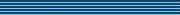### Geographically weighted regression: modelling spatial heterogeneity

Speaker
Martin Charlton
Duration
1:05:02
Date
21 March 2012
Abstract
Geographically Weighted Regression is a technique for exploratory spatial data analysis. In "normal" regression with data for spatial objects we assume that the relationship we are modelling is uniform across the study area - that is, the estimated regression parameters are "whole-map" statistics. In many situations this is not necessarily the case, as mapping the residuals (the differences between the observed and predicted data) may reveal. Many different solutions have been proposed for dealing with spatial variation in these relationships. GWR provides means of modelling such relationships.

This seminar outlines the characteristics of spatial data and the challenges its use poses for analysis, the ideas underpinning geographically weighted regression and details the process of estimating and interpreting the outputs from GWR models. We finish with a brief survey of current issues in GWR and potential future developments.
You should see the video here…
High quality
Fullscreen
Volume